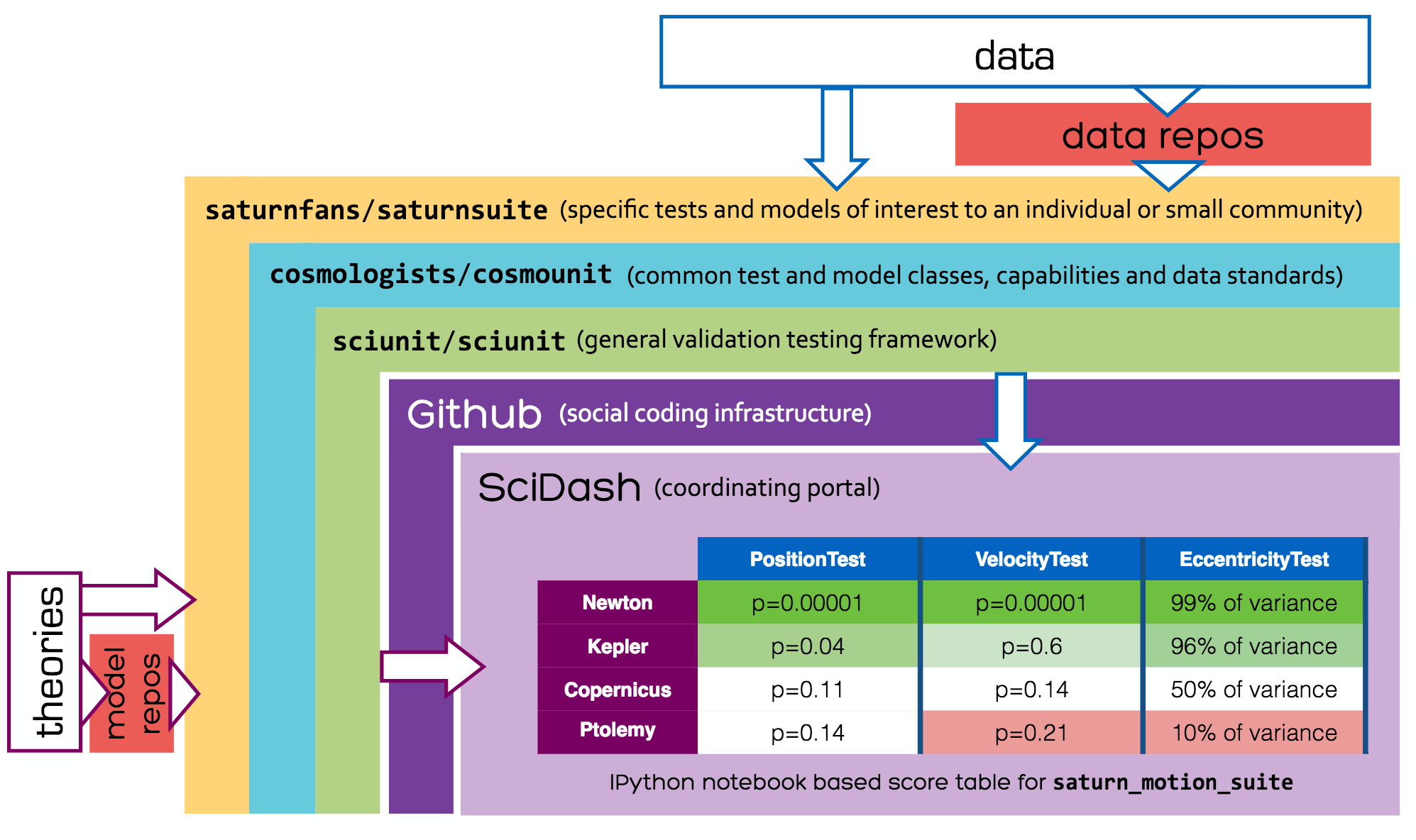# 1. Chapter 1. What is SciUnit?¶

Everyone hopes that their model has some correspondence with reality. Usually, checking whether this is true is done informally.

## 1.1. SciUnit makes this formal and transparent.¶

SciUnit is a framework for validating scientific models by creating experimental-data-driven unit tests.

### 1.1.1. If you are using this file in Google Colab, this block of code can help you install sciunit from PyPI in Colab environment.¶

```!pip install -q sciunit
```

After installation, let’s begin with importing sciunit.

```import sciunit
```

## 1.2. What does testing look like?¶

We’ll start with a simple example from the history of cosmology, where we know that better models displaced their predecessors.
Suppose we have a test suite called “Saturn Suite” that aims to test cosmological models for their correspondence to empirical data about the planet Saturn.
Everything in this example is hypothetical, but once you understand the basic ideas you can visit the documentation for NeuronUnit to see some working, interactive examples from a different domain (neuron and ion channel models).

```from saturnsuite.tests import position_test,velocity_test,eccentricity_test # Examples of test classes.
```

There’s nothing specific to Saturn about position, velocity, or eccentricity. They could apply to any cosmological body.

## 1.3. SciUnit test classes used with in one scientific domain (like cosmology) are located in a discipline-specific library.¶

In this case, the test classes (hypothetically) come from a SciUnit library called CosmoUnit, and are instantiated with data specific to Saturn, in order to create tests specific to a model’s predictions about Saturn:

```'''
saturnsuite/tests.py # Tests for the Saturn suite.
'''
from . import saturn_data # Hypothetical library containing Saturn data.
from cosmounit import PositionTest, VelocityTest, EccentricityyTest # Cosmounit is an external library.
position_test = PositionTest(observation=saturn_data.position)
velocity_test = VelocityTest(observation=saturn_data.velocity)
eccentricity_test = EccentricityTest(observation=saturn_data.eccentricity)
```

This means the test classes are data-agnostic, but the test instances encode the data we want a model to recapitulate.

Next, let’s load some models that aim to predict the cosmological features being assessed by the tests above.

```from saturnsuite.models import ptolemy_model, copernicus_model, kepler_model, newton_model # Examples of models.
```

Ptolemy’s, Copernicus’s, Kepler’s, or Newton’s models could similarly apply to any cosmological body. So these model classes are found in CosmoUnit, and the Saturn Suite contains model instances parameterized to emit predictions about Saturn specifically.

```'''
saturnsuite/models.py # Models for the Saturn suite.
'''
from cosmounit import PtolemyModel, CopernicusModel, KeplerModel, NewtonModel
ptolemy_model = PtolemyModel(planet='Saturn')
copernicus_model = CopernicusModel(planet='Saturn')
kepler_model = KeplerModel(planet='Saturn')
newton_model = NewtonModel(planet='Saturn')
```

In the above each model takes a keyword argument ‘planet’ that determines about what planet the model will make predicitons.

## 1.5. Now we can execute this entire test suite against our models.¶

```from saturn_suite.suites import saturn_motion_suite
saturn_motion_suite.judge([ptolemy_model, copernicus_model, kepler_model, newton_model])
```

The exact output will depend on your preferences (terminal, HTML, etc.) but the figure below illustrates both the results you get (center table) and the relationship between the components listed here.The figure above also refers to SciDash, an in-development portal for accessing public test results, but for the remainder of this tutorial, we will focus on model/test development, execution, and visualization on your own machine.